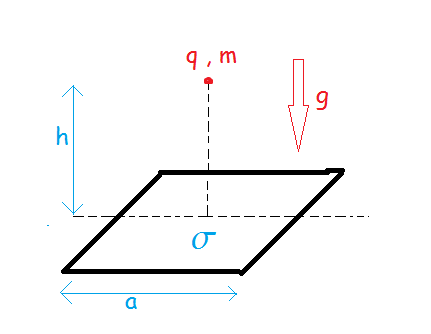# A Charge Oscillating Above A Charged Square Plate.Consider a point charge of mass $m$ and charge $q$ in equilibrium at a height $h$ above a square plate of charge density $\sigma$ and side $a = 2h$ in the presence of gravity in equilibrium. The plate is fixed.

Now, the point charge is given a small displacement in vertical displacement. Find an expression for the time period of its oscillations $T$ in terms of $h$ and $g$, and give the value of $53.72 T$ for $h = 9m$ and $g = 9.8 m/s^2$ to the nearest integer

×